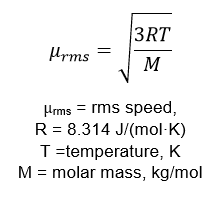# Problem: Consider separate 1.0-L samples of He(g) and UF6(g), both at 1.00 atm and containing the same number of moles. What ratio of temperatures for the two samples would produce the same root mean square velocity?

###### FREE Expert Solution

To solve this problem, recall the formula for root mean square velocity:He(g) and UF6(g) have the same µrms so we can equate the µrms of He(g) and µrms of UF6(g)

Let:      T1 = temperature for He(g)

T2 = temperature for UF6(g)

Determine the Molar mass of He and UF6:

84% (429 ratings)###### Problem Details

Consider separate 1.0-L samples of He(g) and UF6(g), both at 1.00 atm and containing the same number of moles. What ratio of temperatures for the two samples would produce the same root mean square velocity?